# NCERT Solutions Class 5 Mathematics Chapter 10 Tenths And Hundredths

## Chapter 10 Tenths And Hundredths Class 5 Mathematics NCERT Solutions

Class 5 Mathematics students should refer to the following NCERT questions with answers for Tenths And Hundredths in standard 5. These NCERT Solutions with answers for Grade 5 Mathematics will come in exams and help you to score good marks

### Tenths And Hundredths Chapter 10 NCERT Solutions Class 5 Mathematics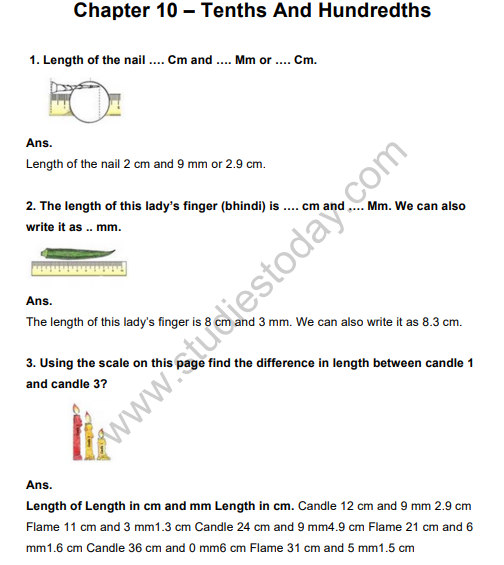4. Our Eyes Get Confused?
Which line is longer? A or B? Measure each line and writes how long it is in centimetres. How good is your guess?Ans. I think line B is longer than A. On Measuring: line (A) = 4.6 cm and Line (B) = 4.6 cm.
My guess is incorrect.

5. Which line is longer? C or D? Measure each line. How good is your guess?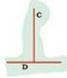Ans. Line (C) appears to be longer than the line (D). On measuring: Line (C) = 3.2 cm and Line (D) = 3.2 cm. My guess in incorrect.

6. At the market

(a) How many paise does a matchbox cost?
Ans. A matchbox costs 50 paise.

(b) How many match-box can be got for Rs. 2.50?
Ans. Five matchboxes can be bought for Rs. 2.50.

(c) How many rupees does the soap cost?
Ans. A soap costs Rs. 8.75.

(d) Arun wanted to buy a soap. He has a five-rupees coin. 2 one-rupee coins and 4 half-rupee coin. Write in rupees what money he will get back?
Ans. Total money with Arun = 1 x Rs. 5 + 2 x Re 1 + 4 x Re. 0.5
= Rs. 5 + Rs. 2 + Rs. 2 = Rs. 9
Cost of a soap = Rs. 8.75
Arun will get back = Rs. 9 – Rs. 8.75
= 900 paise – 875 paise
= 25 paise = Re 0.25

(e) (1) An egg costs two and a half rupees. How much will one and a half dozen cost?
Ans. (1) One dozen =12 pieces
One and a half dozen = (12+6) = 18 pieces
Cost of one egg = Rs. 2.50
Cost of 18 eggs = Rs. (18 x 2.50)
= Rs. 18 x 2 + Rs. 18 x 0.50
= Rs. 36 + Rs. 9 = Rs. 45

(2) How many pens can Kannan buy? How much money is left?
Ans. (2) Money left after purchase of one and a half dozen eggs
= Total money – Money spent
= Rs. 60 – Rs. 45
= Rs. 15
Cost of a pen = Rs. 6.50
Since 1 x Rs. 6.50 = Rs. 6.50, 2 x Rs. 6.50 = Rs. 13
3 x Rs. 6.50 = Rs. 19.50
Money left with Kanna = Rs. 15- Rs. 13 = Rs. 2

(f) The price of two pens is Rs …… Can she buy two pens?
Ans. Price of 1 pen = Rs. 6.50
Price of 2 pens = Rs. (2 x6.50) = Rs. 13
So, she can buy 2 pens with rupees 13.

7. Colourful Design:(a) What part of this sheet is coloured blue?
Ans. (a) part of the given sheet is coloured blue.

(b) Which part of the sheet is green?
Ans. (b) 3/10 part of the given sheet is green.

(c) Which colour covers 0.2 of the sheet?
Ans. (c) Black colour covers 0.2 of the given sheet.

8. Now look at the second sheet. Each strip is divided into 10 equal boxes.
How many boxes are there in all?

(a) Is each box 1/10 part of the sheet?
Ans. (a) Yes, each box is 1/10 part of the sheet.

(b) How many blue boxes are there?
Ans. (b) There are 10 blue boxes.

(c) Is blue equal to 10/100 of the sheet? We saw that blue is also equal to of the sheet. We wrote it as 0.1 of the sheet.
Ans. (c) Yes, blue boxes are equal to 10/100 of the sheet.

(d) Can we say 10/100 = 1/100 = 0.10 = 0.1 ?
Think: Can we write ten paise is 0.1 of a rupee?
Ans. (d) Yes, we can say 10/100 = 1/100 = 0.10 = 0.1.

(e) How many boxes are red? What part of the sheet is this?
15/100 Can we also write it as 0.15 of the sheet?
Ans. There are 15 red boxes. They are 15/100 part of the sheet.
It can also be written as 0.15 of the sheet.

(f) Now 3/100 of the sheet is black. We can say 0. ……. Is black.
Ans. Now 3/100 of the sheet is black. We can say 0.03 sheet is black.

(g) How many white boxes are there in the sheet?
Ans. There are 22 white boxes in the sheet.

(h) What part of the second sheet is white?
Ans. 22/100 part of the second sheet is white.

9. Sports Day The school at Malappuram has its sports day. The first five children in the Long Jump are: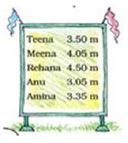(a) Who is the winner in the long jump?
Ans. (a) Rehana was the winner.

(b) Write the names of the I , II and III winners on this stand.
Ans. I is Rehana, II is Meena and III is Teena.

(c) Do you remember that 1 metre = 100 centimetre? So one centimetre is 1/100 of a metre. We also writes 1 cm as ….. m.
Ans. (c) 1 cm as 0.10 m.

10. Money from different countries.
Have you seen any notes or coins used in any other country? Shivam Bank has a chart to show us how many Indian rupees we can get when we change the money of different countries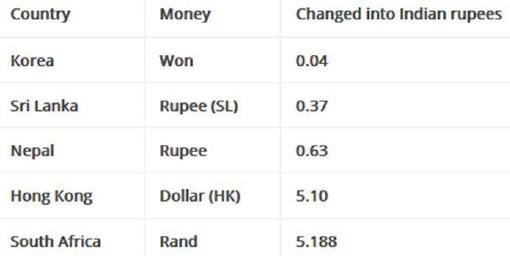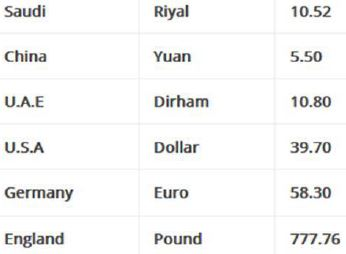(This is the rate on 15-2-2008)
(A) The money of which country will cost the most in Indian Rupees?
Ans. England’s money will cost the most in Indian Rupees.

(B) Mithun’s uncle in America had sent him 10 USA dollars as a gift. Mithun used 350 rupees for a school trip. How much money was left with him.
Ans. Money received by Mithun = 10 USA Dollars
= 10 x Rs. 39.70
= 10 x Rs. 39 + 10 x 70 paise
= Rs. 390 + 700 paise
= Rs. 390 + Rs. 397
Money spend on school trip =Rs. 350
Money left with Mithun = Rs. 397- Rs. 350 = Rs. 47

(C) Majeed’s father is working in Saudi Arabia. He gets 1000, Saudi Riyal as salary. Arun’s father who is working in Sri Lanka gets 2000 Sri Lankan Rupees. Who gets more Indian Rupees.
Ans. Majeed’s father salary = 1000 Saudi Riyal
= 1000 x Rs. 10.52
= 1000 x Rs. 10 + 1000 x 52 paise
= Rs. 10000 + 52000 paise
= Rs. 10000 + Rs. 520 = Rs. 10520
Arun’s father salary = 2000 Sri Lankan Rupees
= 2000 x Rs. 0.37
= 2000 x 37 paise
= 74000 paise = Rs. 740

(D) Leena’s aunty brought a present for her from China. It cost 30 Yuan. Find what it costs in Indian rupees.
Ans. Cost of the present = 30 Yuan
= 30 x Rs. 5.50
= 30 x Rs. 5 + 30 x 50 paise
= Rs. 150 + Rs. 15 = Rs. 165

(E) Astha wants some Hong Kong Dollars and Won.
(1) How many won can Hong Kong Dollars and Won.
Ans. Since 1 won = Re. 0.04
Multiply both sides by 100, we get
100 x 1 Won = 100 x Re. 0.04
= 100 x 4 paise
= 400 paise = Rs. 4
For Rs.4 Astha can have 100 Won
And, for Rs.400, she can have 100 x 100 = 10000 won

(2) How many Hong Kong Dollars can she changes for Rs. 508?
Ans. Since 1 Dollar (HK) = Rs. 5.10
∴ For Rs. 508, Astha can have 99 Dollar and have left with Rs. 508- Rs. 504.90
i.e. Rs. 3.10 in hand.

11. Kiran went shopping with Rs. 200. Look at the bill. The shopkeeper forgot to put the point correctly in the prices. Put the point in the correct place and find out the total amount of the bill.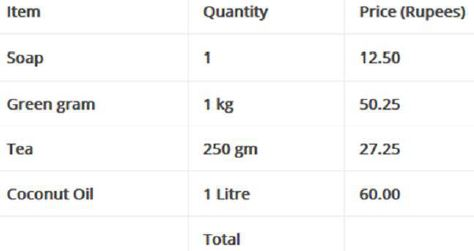Ans.
The correct bill as under:12. Which city is cool?
The temperature in each city was noted at 3pm on 16 January, 2008 as tabulated below:

(a) Which place had the highest temperature at 3 pm? Which place is the coolest at that time?
Ans. (a) Mumbai had the highest temperature at 3 pm. Srinagar had the coolest temperature at 3 pm.

(b) How much higher is the temperature in Mumbai from that in Srinagar?
Ans. (b) The temperature in Mumbai is higher from that in Srinagar by 35.1oC. 8.1oC = 27oC

(c) How many degrees will the temperature need to rise for it to reach 40oC in Thiruvananthapuram?
Ans. (c) There should be a rise of 40oC- 33.5oC = 6.5oC on temperature for it to reach 40oC in Thiruvananthapuram.

(d) How much lower is the temperature of Kolkata from that in Chennai?
Ans. (d) The temperature in Kolkata is lower than in Chennai by 29.9oC – 26.6oC = 3.3oC.

(e) The temperature in these cities was also noted at 3 am on the same day,
Look at the table and answer the questions.

Q. Which place had the lowest temperature at 3 am? Imagine yourself to be there and describe how it would feel?
Ans. Srinagar had the lowest temperature at 3 am. It was very much cold and the water n pipes froze into ice. We had worn heavy woollen clothes and had also taken the help of fire.

Q. What is the difference between the temperatures at 3 pm and 3 am in Chennai?
Ans. The difference between the temperatures at 3 pm and 3 am in Chennai is 29.9oC – 21.1oC = 8.8oC.

 NCERT Solutions Class 5 Mathematics Chapter 1 The Fish Tale
 NCERT Solutions Class 5 Mathematics Chapter 2 Shapes and Angles
 NCERT Solutions Class 5 Mathematics Chapter 3 How Many Squares
 NCERT Solutions Class 5 Mathematics Chapter 4 Parts and Wholes
 NCERT Solutions Class 5 Mathematics Chapter 5 Does it look the same
 NCERT Solutions Class 5 Mathematics Chapter 6 Be my multiply
 NCERT Solutions Class 5 Mathematics Chapter 7 Can you see the pattern
 NCERT Solutions Class 5 Mathematics Chapter 8 Mapping Your Way
 NCERT Solutions Class 5 Mathematics Chapter 9 Boxes And Sketches
 NCERT Solutions Class 5 Mathematics Chapter 10 Tenths And Hundredths
 NCERT Solutions Class 5 Mathematics Chapter 11 Area and its Boundary
 NCERT Solutions Class 5 Mathematics Chapter 12 Smart Charts
 NCERT Solutions Class 5 Mathematics Chapter 13 Ways of Multiply and Divide
 NCERT Solutions Class 5 Mathematics Chapter 14 How Big How Heavy

Tags